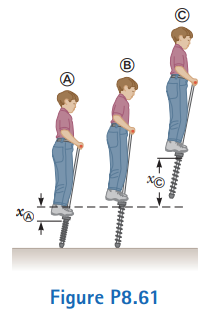# Problem: A child’s pogo stick (Fig. P8.61) stores energy in a spring with a force constant of 2.50 x  104 N/m. At position A (xA = - 0.100  m), the spring compression is a maximum and the child is momentarily at rest. At position B (xB = 0), the spring is relaxed and the child is moving upward. At position C, the child is again momentarily at rest at the top of the jump. The combined mass of child and pogo stick is 25.0 kg. Although the boy must lean forward to remain balanced, the angle is small, so let’s assume the pogo stick is vertical. Also assume the boy does not bend his legs during the motion.(a) Calculate the total energy of the child–stick–Earth system, taking both gravitational and elastic potential energies as zero for x = 0.(b) Determine xC.(c) Calculate the speed of the child at x = 0.(d) Determine the value of x for which the kinetic energy of the system is a maximum.(e) Calculate the child’s maximum upward speed.

###### FREE Expert Solution

In this problem, we'll use the law of conservation of energy.

96% (43 ratings)###### Problem Details

A child’s pogo stick (Fig. P8.61) stores energy in a spring with a force constant of 2.50 x  104 N/m. At position A (xA = - 0.100  m), the spring compression is a maximum and the child is momentarily at rest. At position B (xB = 0), the spring is relaxed and the child is moving upward. At position C, the child is again momentarily at rest at the top of the jump. The combined mass of child and pogo stick is 25.0 kg. Although the boy must lean forward to remain balanced, the angle is small, so let’s assume the pogo stick is vertical. Also assume the boy does not bend his legs during the motion.

(a) Calculate the total energy of the child–stick–Earth system, taking both gravitational and elastic potential energies as zero for x = 0.
(b) Determine xC.
(c) Calculate the speed of the child at x = 0.
(d) Determine the value of x for which the kinetic energy of the system is a maximum.
(e) Calculate the child’s maximum upward speed.Frequently Asked Questions

What scientific concept do you need to know in order to solve this problem?

Our tutors have indicated that to solve this problem you will need to apply the Springs & Elastic Potential Energy concept. You can view video lessons to learn Springs & Elastic Potential Energy. Or if you need more Springs & Elastic Potential Energy practice, you can also practice Springs & Elastic Potential Energy practice problems.

What professor is this problem relevant for?

Based on our data, we think this problem is relevant for Professor Dutta's class at VANDERBILT.

What textbook is this problem found in?

Our data indicates that this problem or a close variation was asked in Physics for Scientists and Engineers - Serway Calc 9th Edition. You can also practice Physics for Scientists and Engineers - Serway Calc 9th Edition practice problems.